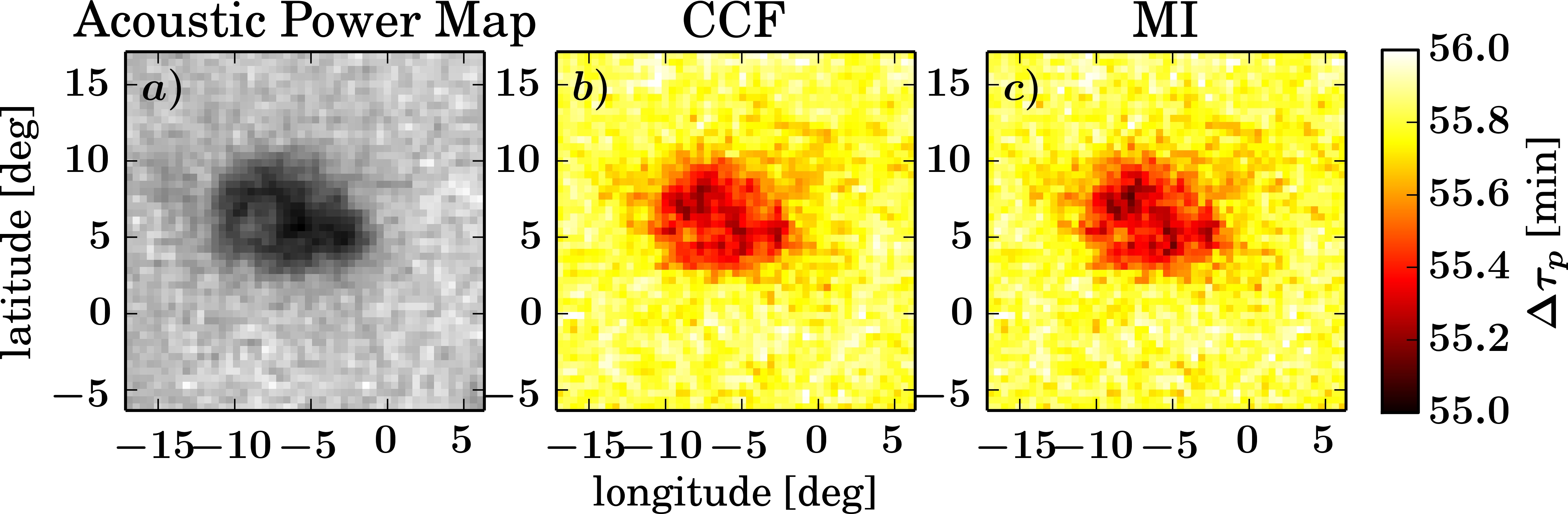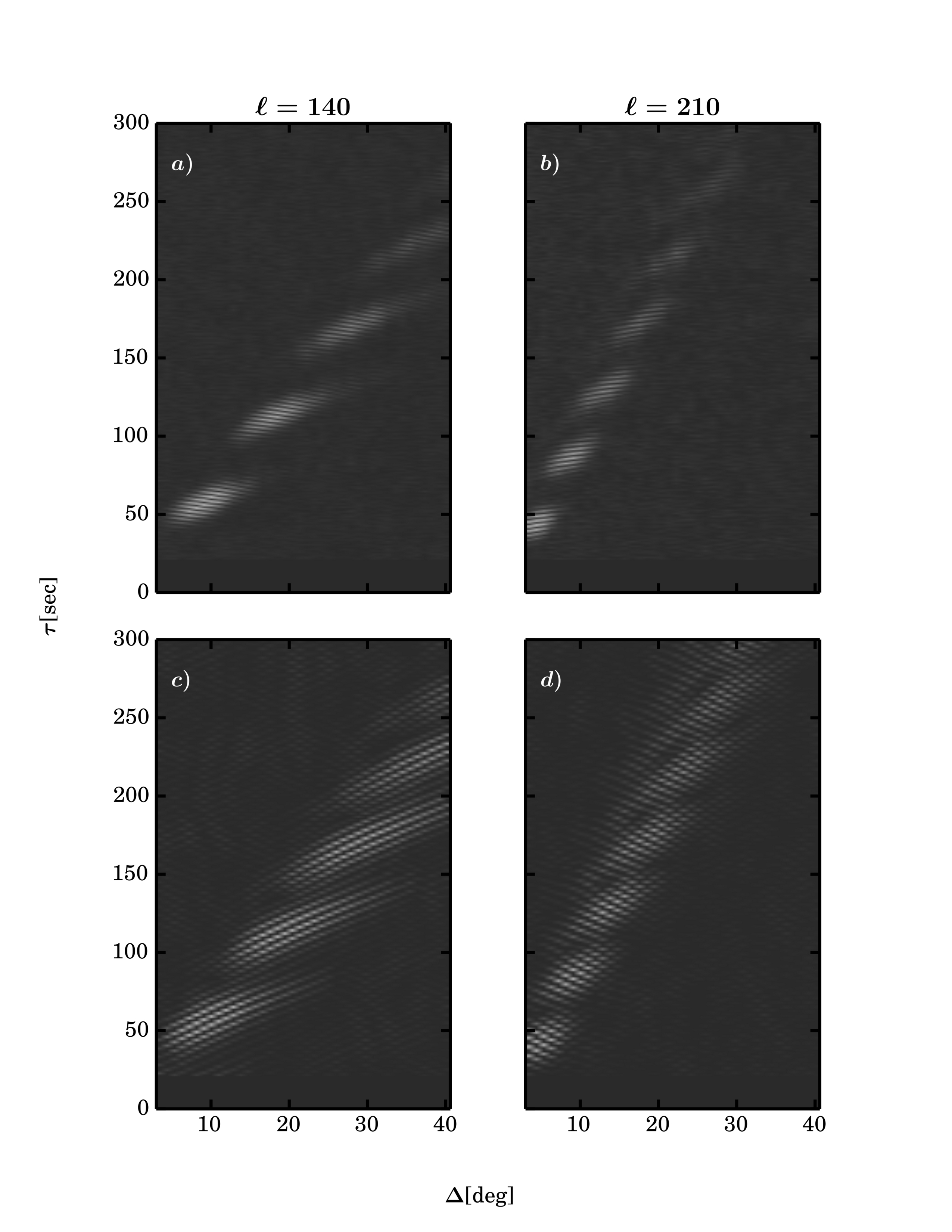# 36. Application of Mutual Information Methods in Time-Distance Helioseismology

Contributed by Dustin Keys. Posted on February 18, 2015

Dustin M. Keys1, Shukur Kholikov1, Alexei A. Pevtsov2
1. National Solar Observatory, Tucson, AZ85719, USA
2. National Solar Observatory, Sunspot, NM88349, USA

For the past 20 years, helioseismologists have had much success in using the cross-correlation, or cross-covariance function (CCF), for computing properties of wave-packets moving through the Sun. The CCF is easy to work with as it is fast computationally and has a simple functional form. However, CCF may not be capturing all the available information on the wave-packets as it is only a measure of the covariance (product of the deviations of two random variables from their means) of the governing probability distribution of two points on the Sun. An alternate measure which can capture more complicated, possibly nonlinear effects is the mutual information or MI, which originates from the theory of communication. Rather than taking the covariance of the joint probability distribution for two points on the Sun, MI takes the expectation of the logarithm of the ratio of the joint distribution to the product of the marginal distributions. Mutual information is closely tied with the entropy, which lends itself to more physical interpretations for MI, though there is still work to be done in this area. For example, in equilibrium situations, the Shannon entropy from which MI is derived and the thermodynamic entropy of the second law of thermodynamics are equivalent, but in non-equilibrium situations the two may differ.

Since Shannon first formalized the theory of information for communications, it has found many uses in a wide variety of other fields ranging from biology to economics, or indeed anywhere stochastic models may be used. Until now, the MI methods were not applied to the field of solar astrophysics.Figure 1. (a) A map of acoustic power showing the structure of AR10484 as a comparison to the travel-time maps. (b) Travel-time deviation map calculated using the CCF method. (c) Travel-time deviation map calculated using the k-nearest neighbor MI method.

To test the applicability of MI to helioseismology, the MI method was applied to data from the Michelson Doppler Imager (MDI) on board of Solar and Heliospheric Observatory (SOHO). The resulting travel-time deviation maps from MI method (Figure 1) are remarkably similar to ones derived by classical CCF method.Figure 2. Time-distance diagrams for spherical harmonic filtered data centered at a)ℓ=140, calculated using MI, b)ℓ=210, calculated using MI, c)ℓ=140, calculated using CCF and d)ℓ=210 calculated using CCF. The diagonal lines at each skip are measures of the phase velocity and it can be seen that the two methods differ in the slope of these lines.

Next, Keys et al.  calculated classical time-distance diagrams and derived the lifetimes of p-modes. Figure 2 shows a comparison between MI time-distance diagrams (a and b) and CCF time-distance diagrams (c and d). These diagrams were calculated from spherical harmonic filtered MDI dopplergrams of the quiet Sun, with a filter centered around ℓ=140 and ℓ =210. Lifetimes calculated for these two spherical harmonic degrees differ by about 10 minutes, with MI giving a longer lifetime as compared with CCF. Further analysis suggests that MI method captures most of the useful information in the joint probability distribution and that the contribution from higher moments (not captured by CCF) is small but nonzero. Close inspection of the time-distance diagram reveals that the behavior of the phase of the wavepacket from these two methods is slightly different. In particular, the phase of the wavelet is changing at a different rate, which one can see by examining the diagonal ridges of the wavepacket at each skip.

This work represents a proof-of-concept approach to MI to demonstrate that the new method (MI) can successfully reproduce the known results of helioseismology found with the CCF, as well as provide a different physical perspective. To get more realistic values of MI would require a cadence and resolution smaller than the dynamic ranges of the Sun. This needs to be explored using data from instruments such as the Solar Dynamic Observatory/Helioseismic and Magnetic Imager (SDO/HMI) and the upcoming Daniel K. Inouye Solar Telescope (DKIST).

### References

 Duvall, T.L. Jr., Jefferies, S.M., Harvey, J.W., Pomerantz, M.A. 1993, Nature, 362, 430
 Shannon, C. 1948, Bell System Technical Journal, 27, 379.
 Jaynes, E.T. 1957, Physical Review, 106(4), 620.
 Keys, D., Kholikov, S., Pevtsov, A.A. 2015, Solar Physics, 290, 659

## 2 comments on “Application of Mutual Information Methods in Time-Distance Helioseismology”

1. Junwei Zhao

Cole, I do not think so. Helioseismology only study things happening on the Sun, and activities on the Sun are believed not to be related with earthquake occurrences.